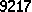This page summarizes a set of video lectures on a subset of computer vision. It is intended for viewers who have an understanding of the nature of images and some understanding of how they can be processed. The course is more like Computer Vision 102, introducing a range of standard and acccepted methods, rather than the latest research advances.

The lectures take the perspective that learning is more effective if learners see how the techniques are used in the context of an application. As real-world applications are generally too complex, we present 6 simplified applications that introduce important methods in 2D image analysis, 3D image analysis, and video analysis.

## I. Course introduction and review

This short set of videos sets the scene by introducing the six example systems. We also review some simple 2D coordinate geometry and polycurve modelling.

## II. Rigid flat part recognition

We use some simple flat rigid shapes to introduce the general principles of model-based recognition, including pose estimation, model matching and verification. We also introduce methods for finding the straight line segments that make up the part's boundary.

## III. Deforming flat part recognition

Many shapes have natural systematic variations, or the parts may come from families where they vary in some standard way. The rigid part recognition approach of the first system is not usually suitable for recognising these new parts. Accordingly, we introduce a technique based on identifying the systematic modes of variation (the Point Distribution Model), which is based on Principalk Component Analysis. We also see an example that extends the variations from a few points to a complete image (the Eigenface method).

## IV. Range data based 3D part recognition

This set of videos extends the rigid 2D part matching algorithm to 3D, which allows us to introduce techniques for acquiring and processing 3D data, including planar patch extraction and 3D pose estimation. We need to also introduce a simple wire-frame modelling system and adapt the 2D Interpretation Tree matching example. We also introduce 3 more examples of least-square parameter estimation algorithms.

## V. Stereo data based 3D part recognition

One approach to obtaining a 3D of a scene is to use 2 cameras in a binocular system, somewhat similar to that used by the human visual system. These lectures look at the geometry and features used for stereo matching. We look at both edge features, which introduces the Canny edge detector, and point features, which introduces the SIFT features. We use the RANSAC algorithm to find straight 2D lines, match them using a set of stereo correspondence constraints, and then use epipolar geometry to compute the 3D position of the lines. Another set of least-square algorithms estimate the pose. Finally, we introduce one of the early approaches to computing a dense depth map by stereo matching of intensity values.

## VI. Detecting and tracking objects in video

We introduce the basic concepts for detection and tracking of objects in videos. Several different moving object detection algorithms are presented that allow detection of objects. These objects are then tracked, using first a Kalman filter, which allows prediction of the future location of the target, and then the Condensation Tracking algorithm, which allows the tracking algorithm to keep multiple hypotheses of the object, and to model expected state changes.

## VII. Persistent tracking and behavior recognition

Once moving objects in videos have been detected and tracked, we can do a variety of things. Here we look at 2 issues: 1) How to connect together sections of tracked people and cars that have been fragmented into separate sections by occlusions, shadows, etc.2) Recognising the current immediate action that a person is making, such as a forehand tennis stroke.

## VIII. Visual ethics

Automated video analysis systems have the potential for unethical use. The note introduces some potentially contentious applications.

## IX. Course Summary

We have looked at a lot of different topics. Here we have a quick summary of the main themes to remind you how much ground you have covered.